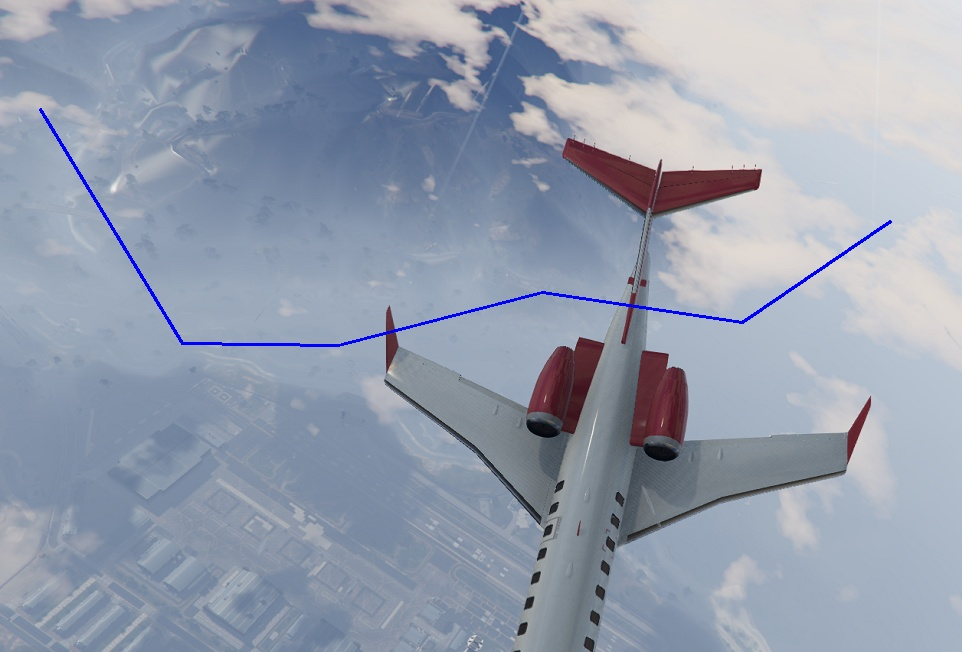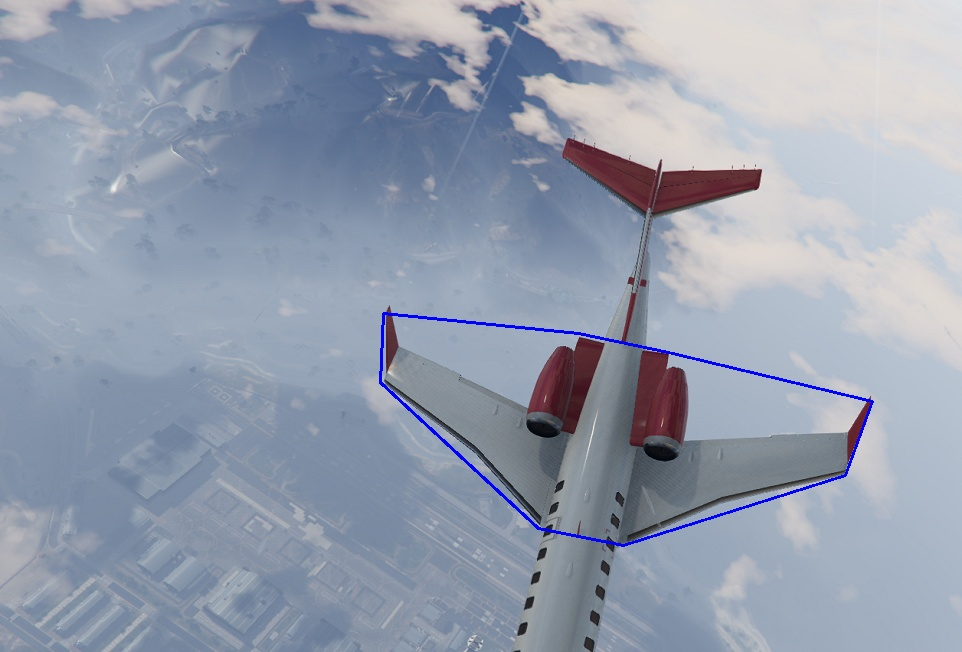Opencv provides different functions to work and draw on images. We can draw lines, polylines etc using opencv methods on different images. In this post we will work on creating multiple connected lines(polylines) and polygons.

In this post we worked on drawing lines on images using cv2.line() and cv2.arrowedLine() method. But if we have more than two points and we need to draw a lot of lines between two points, for this cv2 line method can be used but for performance we can use polylines method from opencv.

### Polylines

Polylines create lines for a list of points. It requires a list of x,y points and can create lines between all points in that list. It requirest following values to work with.

• image
• Points array - Point array should be int32 and have shape of (noPoints, 1, 2)
• isClosed - Check if it is closed
• Color - Color of polyline
• Thickness - Thickness of line
• Line Type - Type of line could be `FILLED``LINE_4``LINE_8``LINE_AA`View More

Now we can read an image using opencv and draw polyline using a list of points.

``````# import modules and read image file
import cv2
import numpy as np

# List of points
points = [[40, 109], [182, 343], [338, 345], [542, 292], [742, 322], [890, 221]]
# convert to numpy array and reshape
points = np.array(points)
points = points.reshape((-1, 1, 2))

# color, thickness and isClosed
color = (255, 0, 0)
thickness = 2
isClosed = False

# drawPolyline
image = cv2.polylines(image, [points], isClosed, color, thickness)

# show image
cv2.imshow("image", image)
cv2.waitKey(0)
cv2.destroyAllWindows()``````

This draws a line for given points and it shows as following.We can also draw closed polyline as polygon, we just change is closed to True. When we set isClosed to true, it connects first and last point in our points array with a line.

``````# new points for polygon
points = [[383, 313], [380, 382], [538, 528], [623, 545], [845, 475], [872, 401], [668, 352], [578, 333]]
# create and reshape array
points = np.array(points)
points = points.reshape((-1, 1, 2))

# Attributes
isClosed = True
color = (255, 0, 0)
thickness = 2

# draw closed polyline
image = cv2.polylines(image, [points], isClosed, color, thickness)``````

This draws a polygon for points and we can view that it automatically connected first and last point with a line.For more details on cv2 drawing methods, check opencv-python documentation.

https://docs.opencv.org/4.x/dc/da5/tutorial_py_drawing_functions.html